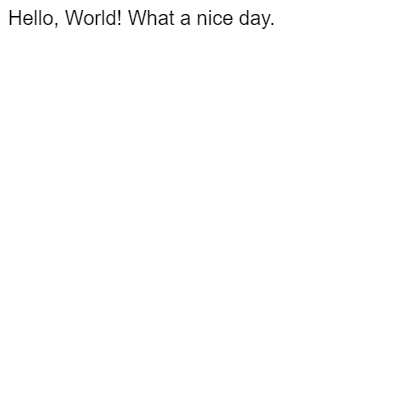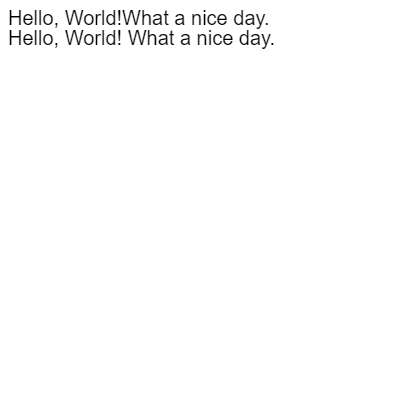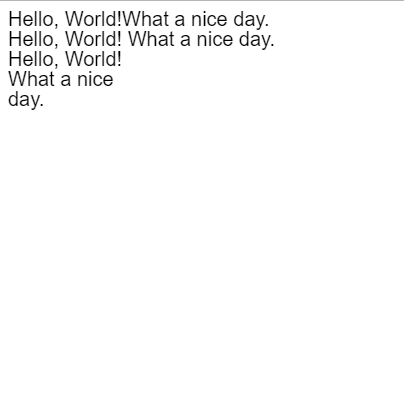# HTML5 canvas 绘制的文字如何换行？

HTML5 里的 canvas 元素是一个图形容器，我们可以通过 JavaScript 来控制它绘制各种文本和图像。

example.html

<!doctype html>
<html>
<meta charset="UTF-8">
<title>Example</title>
<body>
<canvas id="canvas" height="400" width="400"></canvas>
</body>
<script src="main.js"></script>
</html>



main.js

var c = document.getElementById("canvas");
var context = c.getContext("2d");

function draw(){
context.font = "20px Arial";
context.fillStyle = "black";
context.textBaseline = "middle";
context.fillText("Hello, World!\nWhat a nice day.",0,10);
}

draw();function draw(){
context.font = "20px Arial";
context.fillStyle = "black";
context.textBaseline = "middle";
context.fillText("Hello, World!What a nice day.",0,10);
context.fillText("Hello, World!\nWhat a nice day.",0,30);
}/****绘制自动换行的字符串****/

function drawText(t,x,y,w){

var chr = t.split("");
var temp = "";
var row = [];

context.font = "20px Arial";
context.fillStyle = "black";
context.textBaseline = "middle";

for(var a = 0; a < chr.length; a++){
if( context.measureText(temp).width < w ){
;
}
else{
row.push(temp);
temp = "";
}
temp += chr[a];
}

row.push(temp);

for(var b = 0; b < row.length; b++){
context.fillText(row[b],x,y+(b+1)*20);
}
}


drawText("Hello, World!What a nice day.",0,30,110);